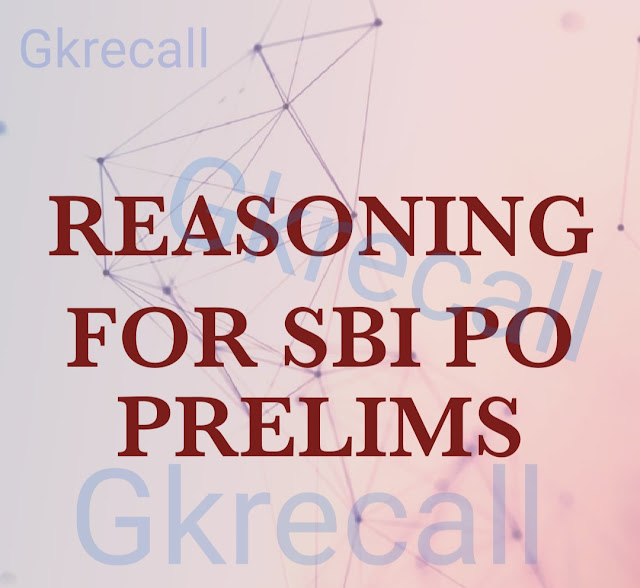# gkrecall

We brings you daily Current affairs, daily Current Affairs Quiz, weekly Current Affairs, weekly Current Affairs Quiz to enhance your preparation for upcoming exams. We also provide articles related to quant, reasoning, English, ssc etc .

## BreakingWe bring you the complete and important daily Reasoning  to achieve more marks in Banking, Insurance, UPSC, SSC, CLAT, Railways and all other competitive Exams. We prepare it based on our daily current affairs.Hope you like it.

START QUIZ
Directions (1 –5): Study the following information carefully to answer the given questions.
If ‘A () B’ means ‘B is the mother of A’
If ‘A of B’ means ‘B is the father of A’
If ‘A ÷ B’ means ‘B is the sister of A’
If ‘A × B’ means ‘B is the brother of A’
If ‘A + B’ means ‘B is the daughter of A’
If ‘A − B’ means ‘B is the son of A’

1. If ‘T – P ÷ Q’ is given, then how is Q related to T?
A. Nephew
B. Brother
C. Aunt
D. Uncle
E. Daughter

2. What does ‘L () M ÷ N’ means?
A. M is the sister of N.
B. N is the sister of M.
C. M is the niece of N.
D. N is the brother of M.
E. Both (1) and (2)

3. If ‘P of Q + R’ is given, then which of the following is true?
A. P and R are sisters to each other.
B. P is the mother of Q.
C. R is the sister of P.
D. Q is the mother of R.
E. P is the brother of R.

4. If ‘Y × Z () K’ is given, how is Z related to K?
A. Z and Y are brothers to each other.
B. K is the aunt of Y.
C. Z is the son of K.
D. Y is the son of K.
E. Y is the niece of Z.

5. If ‘S × J + T’ is given then which of the following is true?
A. T is the aunt of S.
B. T is the niece of S.
C. S is the uncle of T.
D. S is the brother of J.
E. S is the aunt of T.

Solution-
1. Option E
2. Option E
3. Option C
4. Option C
5. Option B
Directions (6-10): In each of the following questions some statements are given and these statements are followed by three conclusions numbered I, II and III. You have to take the given statements to be true even if they seem to be at variance from commonly known facts. Read the conclusions and then decide which of the given conclusions logically follow from the given statements, disregarding commonly known facts.
6.Statements:
Only a few pen are ink
No ink are eraser
Only Pencil are eraser
No pencil is ink
Conclusions:
I) Some eraser can be ink
II) All eraser can never be ink
III) All pen are ink is a possibility
A. Only I follows
B. Only II follows
C. Only III follows
D. Only I and II follow
E. None of these
7. Statements:
Some lily are rose
All rose are lotus
No lotus is begonia
Conclusions:
I)Some rose are not begonia
II) All lily are lotus is a possibility
III) Few lotus can be rose
A. All follow
B. Only I and II follow
C. Only III follows
D. Only II follows
E. None of these
8. Statements:
All oceans are seas
All seas are river
No seas is canal
Conclusions:
I) Some canals are not river
II) Some rivers are not ocean
III) Some oceans can be canal
A. None follows
B. Only I and II follow
C. All follow
D. Only II follow
E. None of these
9. Statements:
Only a few circle are square
Some square are hexagon
No square is triangle
Conclusions:
I) All circle can never be triangle
II) Some hexagon can be circle
III) All square are circle is a possibility
A. All follow
B. Only I and III follow
C. Only I and II follow
D. Only III follows
E. None of these
10. Statements:
All cakes are candy
No candy is cream
Some creams are orange
Conclusions:
I)Some cakes are orange is a possibility
II)Some cream are not candy
III) All cakes are orange is a possibility
A. All follow
B. None follows
C. Only I and II follow
D. Only II and III follow
E. None of these
Solution-
6. Option B
7. Option B
8. Option A
9. Option A
10. Option D